# 单智能体强化学习算法

## Generalization to New Actions in Reinforcement Learning

Posted by MY on August 10, 2020

## 一、问题

• agent需要观察action的影响或者与action实际交互来理解新的action
• 如何从对action影响的观察中提取出有效的信息

## 二、解法

• 对action observation学习表征，得到一个action representation space；
• 将action representation space输入到策略网络中并使用RL进行训练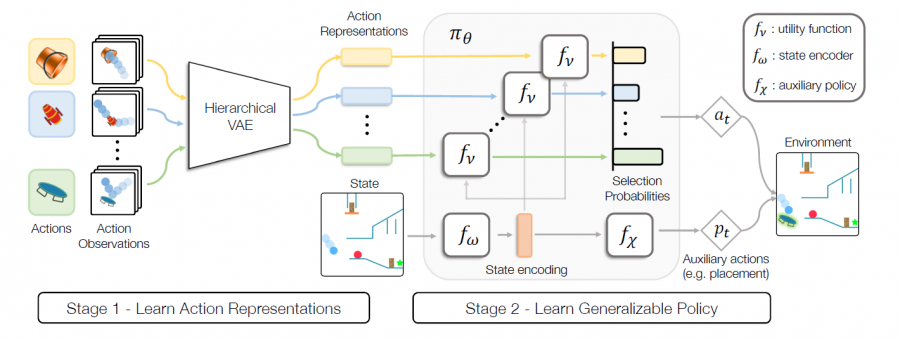### 2.1 问题定义

$R=\mathbb{E}_{\mathcal{A} \subset \mathbb{A}^{\prime}, a \sim \pi(a \mid s, \mathcal{A})}\left[\sum_{t=1}^{T} \gamma^{t-1} \mathcal{R}\left(s_{t}\right)\right]$

### 2.2 无监督动作表征

\begin{aligned} \mathcal{L}=& \sum_{\mathcal{O} \in \mathbb{O}}\left[\mathbb{E}_{q_{\phi}(c \mid \mathcal{O})}\left[\sum_{o \in \mathcal{O}} \mathbb{E}_{q_{\psi}(z \mid o, c)} \log p(o \mid z, c)\right.\right.\\ &\left.\left.-D_{K L}\left(q_{\psi} \| p(z \mid c)\right)\right]-D_{K L}\left(q_{\phi} \| p(c)\right)\right] \end{aligned}

### 2.3 自适应策略网络

$\pi\left(a_{i} \mid s, \mathcal{A}\right)=\frac{e^{f_{\nu}\left[c_{i}, f_{\omega}(s)\right]}}{\sum_{j=1}^{k} e^{f_{\nu}\left[c_{j}, f_{\omega}(s)\right]}}$

### 2.4 泛化目标与训练流程

• 对动作空间进行降采样
• 增加随机策略的熵$\mathcal{H}\left[\pi_{\theta}(a \mid s)\right]$进行正则化辅助
• 在验证集上验证选表现最好的模型

$\max _{\theta} \mathbb{E}_{\mathcal{A} \subset \mathbb{A}, a \sim \pi_{\theta}(. \mid s, \mathcal{A})}\left[R(s)+\beta \mathcal{H}\left[\pi_{\theta}(a \mid s, \mathcal{A})\right]\right]$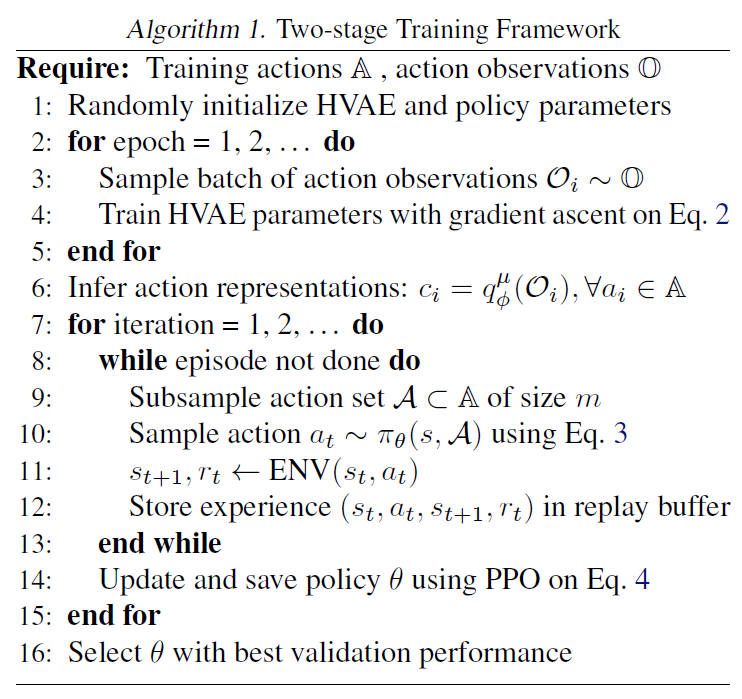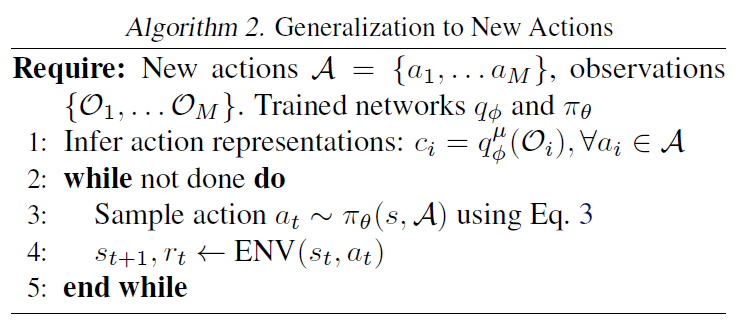## 三、实验内容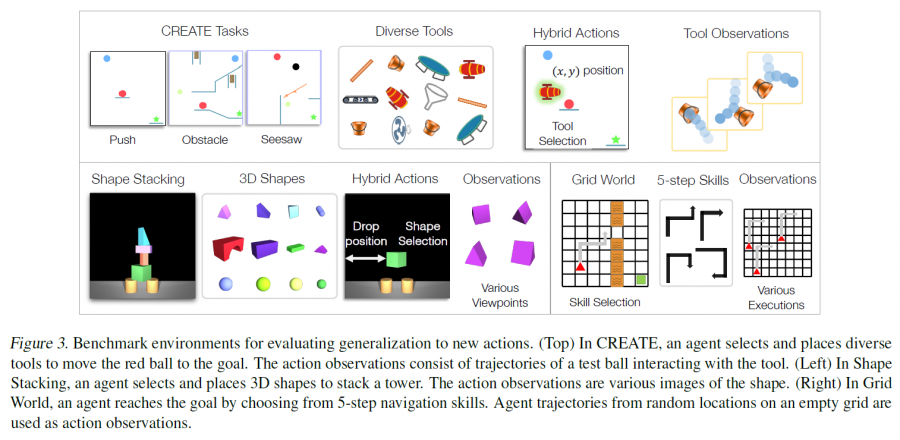HVAE可以提取出action observation中的有效信息：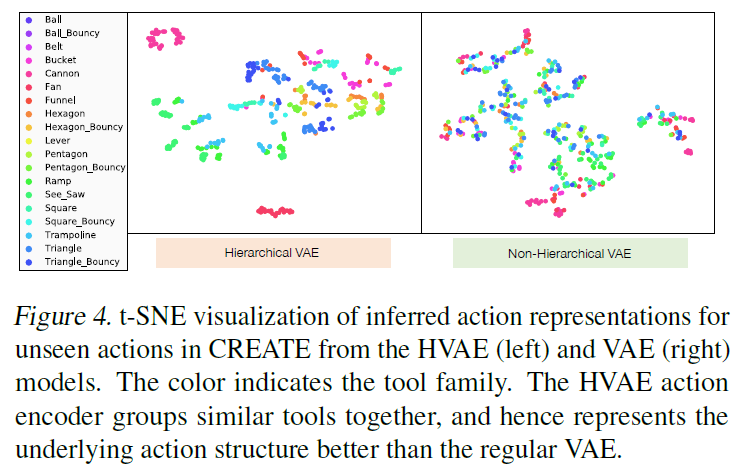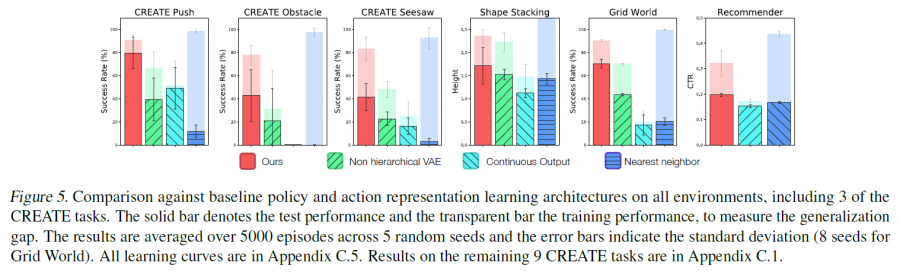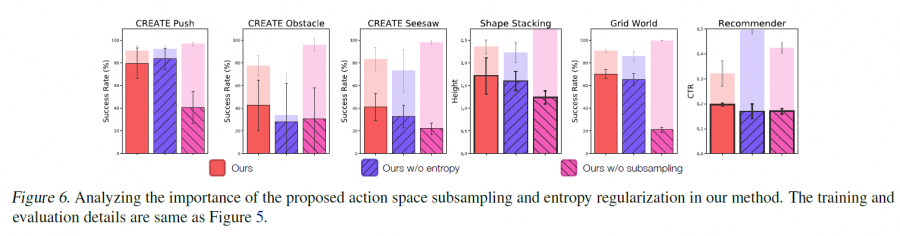## 五、优点

• 第一个在动作集上进行泛化研究的工作
• 提出了一个很酷的benchmark
• 使用HVAE对动作进行表征的方式值得学习
• 在动作集上进行泛化是一个具有现实意义的工作

##### Share